International Journal of Astrophysics and Space Science
Volume 3, Issue 4, August 2015, Pages: 65-69

A Derivation of the Etherington’s Distance-Duality Equation

Yuri Heymann

Athens Institute for Education and Research, Physics, Geneva, Switzerland, Greece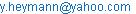Yuri Heymann. A Derivation of the Etherington’s Distance-Duality Equation. International Journal of Astrophysics and Space Science. Vol. 3, No. 4, 2015, pp. 65-69. doi: 10.11648/j.ijass.20150304.13

Abstract: The Etherington's distance-duality equation is the relationship between the luminosity distance of standard candles and the angular-diameter distance. This relationship has been validated from astronomical observations based on the X-ray surface brightness and the Sunyaev-Zel'dovich effect of galaxy clusters. In the present study, we propose a derivation of the Etherington's reciprocity relation in the dichotomous cosmology.

Keywords: Etherington, Distance Duality, Dichotomous Cosmology

1. Introduction

The Etherington's distance-duality equation was introduced in 1933 . Etherington mentioned this equation was proposed by Tolman as a way to test a cosmological model. Ellis proposed a proof of this equation in the context of Riemannian geometry [2-3]. A quote from Ellis : "The core of the reciprocity theorem is the fact that many geometric properties are invariant when the roles of the source and observer in astronomical observations are transposed". This statement is fundamental in the reciprocity theorem as shown here in the derivation of the theorem in the dichotomous cosmology. While the proof of the Etherington's distance duality in the context of Riemannian geometry is tedious, the derivation in the dichotomous cosmology is straightforward. As a reminder, the dichotomous cosmology [4-5] consists of a static matter universe with an expanding luminous world. One needs to imagine a cube of light expanding, in a space where galaxies do not recede from each other.

Fortunately, the Etherington's distance-duality equation, which is a crucial relationship in cosmology, can be verified from astronomical observations. While the luminosity distance is measured from supernova observations, the angular-diameter distance is determined from the X-ray surface brightness and the Sunyaev-Zel'dovich effect  of galaxy clusters . In , the authors found that the ratio between the two distances DL for the luminosity distance and DA for the angular-diameter distance, defined as h = DL/DA(1+z)2 is bound to be h = 1.01 +/- 0.07 at 68% c.l. Similar results were obtained in [9-10], where no significant violation of the distance-duality relationship was found. In , the authors tested the cosmic distance duality for different galaxy cluster samples. The study  is focused on analytical expressions for the deformation of the distance duality in terms of the cosmic absorption parameter. The reciprocity theorem is considered to be true when photon number is conserved, gravity is described by a metric theory with photons traveling on unique null geodesics . Any violation of the distance duality would be attributed to exotic physics.

Following the introduction in section 1, the distance measurements are derived in section 2. To derive the Etherington's reciprocity theorem in the dichotomous cosmology, we first need the distance measurements, which may be derived from the tired-light paradigm (section 2.1) or from expanding metrics (section 2.2). Both derivations lead to the same equations. In section 2.3, we derive the Etherington's distance duality using our distance measurements. In section 3, we provide a brief explanation of the method used to estimate the angular-diameter distance from X-ray surface brightness measurements and the Sunyaev-Zel'dovich effect. In section 4, we present the line of thought of the dichotomous cosmology. Finally, we offer our conclusion in section 5.

2. Derivation of the Distance Measurements

Let us recall the derivation of the distance measurements in the dichotomous cosmology.

When a photon loses energy during its travel in space, the wavelength of light is stretched, and because the number of cycles of the light wave is conserved, an expansion of the luminous world is produced. As a consequence of this stretching of light, the velocity of the light wavefront increases during its travel (Fig. 1). According to special relativity, the speed of light is invariable. Hence, in order to maintain the light wavefront at the speed of light, the model introduces a time contraction between the emission point and the observer. The study  mentions a time dilation; in order to rectify this, the model is based on a time contraction in the arrow of time.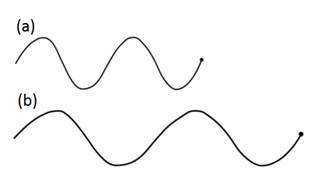Figure 1. Light wavefront. Where (a) is the light wavefront without stretching, and (b) with stretching. We can see that in (b) the light wavefront is going faster than in (a).

Considering that photons lose energy as light gets stretched, the following equation is obtained: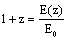(1)

where E(z) is the photon energy when emitted, E0 is the photon energy at time of observation, and z is the redshift.

A simple decay law of the photon energy is considered: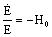(2)

where H0 is the Hubble constant.

Therefore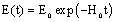(3)

and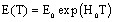(4)

where t is the time which is equal to zero at the time of observation, and T the light travel time of the source from the observer.

A set of two transformations is considered: first a time-variable light wavefront to accommodate the expansion of the luminous world, and second a time contraction to maintain the light wavefront at the speed of light.

2.1.1. Light Wavefront with Respect to the Source

The light wavefront velocity before time contraction is expressed as follows: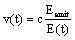(5)

where Eemit is the photon energy when emitted, and E(t) the photon energy at time t.

To maintain the light wavefront at the speed of light, the following time contraction is applied: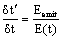(6)

Hence, the light travel time with respect to the source is: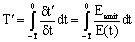(7)

Introducing (3) in the previous equation and integrating, we get: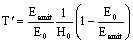(8)

Introducing (1) in the previous equation, we get: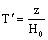(9)

which is the light travel time measurement for the luminosity distance.

2.1.2. Light Wavefront with Respect to the Observer

The light wavefront velocity before time contraction is expressed as follows: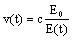(10)

To maintain the light wavefront at the speed of light, the following time contraction is applied: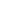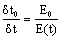(11)

Hence, the light travel time with respect to the observer is: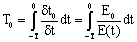(12)

Introducing (3) in the previous equation and integrating, we get: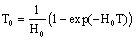(13)

Introducing (4) in the previous equation, we get: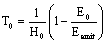(14)

Introducing (1) in the previous equation, we get: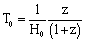(15)

which is the light travel time measurement for the actual distance.

2.2. Derivation from Expanding Metrics

In the dichotomous cosmology, the luminous world is expanding; therefore, we can derive the distance measurements using expanding metrics.

2.2.1. Luminosity Distance

The luminosity distance is the distance measured from the luminosity of standard candles. Supernovae Ia are considered standard candles, meaning they all have the same absolute brightness when they explode. From their apparent brightness, we can deduce the luminosity distance, because the brightness diminishes proportionally to the inverse of the distance squared. The formula used to measure the luminosity distance is the distance modulus equation.

For considering a photon travelling away from the center of a supernova, the luminosity distance is calculated as follows: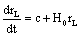(16)

where rL is the luminosity distance, H0 the Hubble constant, and c the speed of light.

By integrating this equation between 0 and T, we get: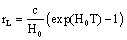(17)

Because da/dt = H0a, we get dt = da/H0a, where a is the scale factor. In addition, the relationship between the scale factor and the redshift is given by the cosmological redshift equation (1 + z) = 1/a , where the scale factor is equal to one at present time. Hence, the light travel time versus redshift is as follows: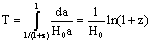(18)

Equations (17) and (18) yield: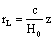(19)

which is identical to (9) with rL = cT’.

2.2.2. Euclidean Distance

A measurement of the distance is obtained by calculating the corresponding distance if there were no expansion, which we call the Euclidean distance. Let us introduce y to this distance measurement. By considering a photon moving towards the observer, we get: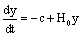(20)

By setting time zero at a reference Tb in the past, we get: t = Tb - T; therefore, dt = -dT (where T is the light travel time when looking at a source into the past). Hence: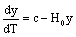(21)

with boundary condition y(T = 0) = 0.

Integrating this equation between 0 and T, we get: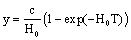(22)

By substitution of (18) into (22), we get :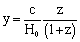(23)

which is identical to (15) with y = cT0.

2.3. Etherington’s Distance Duality

From (19) and (23), we get: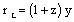(24)

The angular-diameter distance dA of an object is defined in terms of x, the object's actual size, and q, the angular size of the object as viewed from earth. The equation is as follows: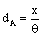(25)

Because of the expansion of the luminous world, the apparent size of celestial objects is stretched by a factor (1+z), and the apparent angular size is increased by the same factor. Hence, the relationship between the actual distance y and the angular-diameter distance is as follows: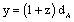(26)

Equations (24) and (26) yield: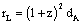(27)

which is the Etherington's distance-duality relationship. We have just derived the Etherington's reciprocity theorem.

3. Method

It is worthwhile to provide a brief explanation of the method used to validate the Etherington’s distance-duality equation based on astronomical observations. The luminosity distance is measured from supernova observations using the distance modulus and is related to the redshift. The challenge is to measure the angular-diameter distance because we don’t know the actual size of astronomical objects. This has been done for galaxy clusters using X-ray surface brightness measurements and the Sunyaev-Zel'dovich effect. Galaxy clusters contain large quantities of hot and ionized gas at temperatures between 10 to 100 megakelvins. This hot gas radiates in the X-ray domain through bremsstrahlung, or radiation produced by the deceleration of a charged particle when deflected by another charged particle. This intra-cluster gas distorts the cosmic microwave background radiation (CMBR) through the so-called Sunyaev-Zel’dovich effect: the inverse Compton interaction of photons which receive an energy boost when colliding with high energy free electrons. This decreases the CMBR brightness at low frequencies but increases it at high frequencies. The drop in temperature or brightness of the CMBR spectrum in the Rayleigh-Jeans region due to the Sunyaev-Zel’dovich effect is a function of electron temperature and density. The X-ray surface brightness is a function of the volume of the cluster and electron temperature and density. Using both measures, we can therefore eliminate the electron density term and estimate the size of the cluster. Finally, we compute the angular-diameter distance using the size of the cluster and angular size as shown in (25). The details of the method and quantitative aspects are described in [14-15].

4. Interpretation

The dichotomous cosmology is in line with the school of thought of the Greek philosopher Democritus. Born around 460 B.C., Democritus was a materialist philosopher disciple of Leucippus. Both held that everything is composed of atoms, the smallest particle of a substance, which interact with each other and lie in empty space. In the dichotomous cosmology there is no need for dark energy or other exotic substances, and the universe consists of atoms and vacuum.

The dichotomous cosmology is in contradiction with the big bang theory. In the big bang theory the universe is expanding, whereas in the dichotomous cosmology the universe is static. The three pillars of the big bang are respectively, the expansion of the universe according to Hubble’s law, the discovery of the microwave background radiation, and the relative abundances of light elements. The dichotomous cosmology challenges the first pillar of the big bang. A consequence of our theory is that the age of the universe is indefinite. In the big bang theory, the age of the universe, is defined by the moment when all the universe was confined in one point - the big bang singularity, which is estimated to have occurred around 13.7 billion years ago. In the dichotomous cosmology, we cannot define a beginning of time. Hubble time, which is the inverse of the Hubble constant, becomes the maximum distance that light can travel in the universe.

5. Conclusion

The Etherington's distance-duality equation, which relates the luminosity distance of standard candles to the angular-diameter distance, is a crucial relationship in cosmology. Although the Etherington's reciprocity theorem is considered to be peculiar to cosmological models based on Riemannian geometry, in the present study we propose a new derivation of this relationship in the dichotomous cosmology. This derivation is straightforward and follows naturally from the dichotomous cosmology. Today, the Etherington's reciprocity theorem is considered established and has been verified using astronomical observations based on X-ray surface brightness and the Sunyaev-Zel'dovich effect of galaxy clusters.

References

1. I.M.H. Etherington, "LX. On the Definition of Distance in General Relativity", Philosophical Magazine, Vol. 15, S. 7 (1933), pp. 761-773.
2. G.F.R. Ellis, "Relativistic cosmology", Proceedings of the 47th International School of Physics "Enrico Fermi", edited by R.K. Sachs (Academic Press, New York and London), Vol. 15 (1971), pp. 104-182.
3. G.F.R. Ellis, "On the Definition of Distance in General Relativity: I.M.H. Etherington (Philosophical Magazine ser. 7, vol. 15, 761 (1933))", General Relativity and Gravitation, Vol. 39 (2007), pp. 1047-1052.
4. Y.Heymann, "The Dichotomous Cosmology with a Static Material World and Expanding Luminous World", Progress in Physics, Vol. 10, Issue 3 (2014), pp. 178-181.
5. Y.Heymann, "A Monte Carlo Simulation Framework for Testing Cosmological Models", Progress in Physics, Vol. 10, Issue 4 (2014), pp. 217-221.
6. R.A. Sunyaev, and Ya.B. Zel’dovich, "The Observation of Relic Radiation as a Test of the Nature of X-Ray Radiation from the Clusters of Galaxies", Comments on Astrophysics and Space Physics, Vol. 4 (1972), pp.173-178.
7. J. Silk, and S.D.M. White, "The Determination of qo Using X-Ray and Microwave Measurements of Galaxy Clusters", The Astrophysical Journal Letters, Vol. 226 (1978), L103.
8. F. Bernardis, E. Giusarma, and A. Melchiorri, "Constraints on Dark Energy and Distance Duality from Sunyaev-Zel’dovich Effect and Chandra X-Ray Measurements", International Journal of Modern physics D, Vol. 15, No. 5 (2006), pp. 759-766.
9. J.-P. Uzan, N. Aghanim, and Y. Mellier, "Distance Duality Relation from X-Ray and Sunyaev-Zel’dovich Observations of Clusters", Physical Review D, Vol. 70, 083533(2004).
10. R. Nair, S. Jhingan, and D. Jain, "Cosmic Distance Duality and Cosmic Transparency", Preprint, arXiv: astro-ph/1210.2642(2012).
11. R.S. Gonçalves, R.F.L. Holanda, and J.S. Alcaniz, "Testing the Cosmic Distance Duality with X-Ray Gas Mass Fraction and Supernovae Data", Monthly Notice Letters of the Royal Astronomical Society, Vol. 420, Issue 1 (2012), L43-L47.
12. J.A.S. Lima, J.V. Cunha, and V.T. Zanchin, "Deformed Distance Duality Relations and Supernova Dimming", The Astrophysical Journal Letters, Vol. 742, No. 2 (2011), L26.
13. B.A. Bassett,and M. Kunz,"Cosmic Distance-Duality as a Probe of Exotic Physics and Acceleration", Physical Review D, Vol. 69, 101305 (2004).
14. M. Birkinshaw, J.P. Hughes, and K.A. Arnaud, "A Measurement of the Value of the Hubble Constant from the X-Ray Properties and the Sunyaev-Zel'dovich Effect of Abell 665", The astrophysical Journal, Vol. 379 (1991), pp. 466-481.
15. Y. Inagaki, T. Suginohara, and Y. Suto, "Reliability of the Hubble-Constant Measurement Based on the Sunyaev-Zel'dovich Effect", Publications of the Astronomical Society of Japan, Vol. 47 (1995), pp. 411-423.

 Contents 1. 2. 2.1. 2.2. 2.3. 3. 4. 5.
Article ToolsAbstractPDF(193K)